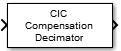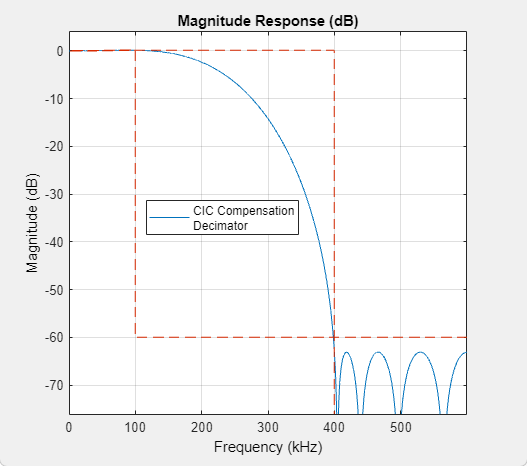# CIC Compensation Decimator

Compensate for CIC filter using FIR decimator

•Libraries:
DSP System Toolbox / Filtering / Multirate Filters

## Description

The CIC Compensation Decimator block uses an FIR polyphase decimator as the compensation filter. CIC compensation decimators are multirate FIR filters that you cascade with CIC decimators to mitigate the drawbacks of the CIC filters.

CIC decimation filters are used in areas that require high decimation. These filters are popular in ASICs and FPGAs, since they do not have any multipliers. CIC filters have two drawbacks:

• CIC filters have a magnitude response that causes a droop in the passband region. This magnitude response is:

$abs{\left(\frac{\mathrm{sin}\left(M\frac{\omega }{2}\right)}{\mathrm{sin}\left(\frac{\omega }{2}\right)}\right)}^{n}$

• M — Differential delay

• n — Number of stages

• ω — Normalized angular frequency

• CIC filters have a wide transition region.

The compensation decimator filters have an inverse passband response to correct for the CIC droop, and they have a narrow transition width.

This block brings the capabilities of the `dsp.CICCompensationDecimator` System object™ to the Simulink® environment.

## Ports

### Input

expand all

Specify the data input as a vector or a matrix.

The block treats a P-by-Q input matrix as Q independent channels, decimating each channel over the first dimension.

When you input a variable-size signal (frame length changes during simulation), the frame length of the signal P can be arbitrary, that is, the input frame length does not have to be a multiple of the decimation factor. When you input a fixed-size signal (frame length does not change during simulation), the frame length can be arbitrary only when you select the Allow arbitrary frame length for fixed-size input signals parameter.

Data Types: `single` | `double` | `int8` | `int16` | `int32` | `int64` | `fixed point`

### Output

expand all

Filtered and downsampled signal, returned as a vector or matrix.

The output signal dimensions depend on the input signal dimensions, the decimation factor of the compensator, and whether you select the Allow arbitrary frame length for fixed-size input signals parameter.

This table provides more details on the dimensions of the output signal when you input a fixed-size input signal and a variable-size input signal.

Input Signal SizeInput Signal DimensionsOutput Signal Dimensions
Fixed

P-by-1 column vector, where P is a multiple of the decimation factor M

(P/M)-by-1

Fixed

P-by-1 column vector, where P is not a multiple of the decimation factor M

`ceil`(P/M)-by-1 when you select Allow arbitrary frame length for fixed-size input signals.

If you do not select Allow arbitrary frame length for fixed-size input signals, the block errors.

Fixed

P-by-Q matrix, where P is a multiple of the decimation factor M

(P/M)-by-Q

Fixed

P-by-Q matrix, where P is not a multiple of the decimation factor M

`ceil`(P/M)-by-Q when you select Allow arbitrary frame length for fixed-size input signals.

If you do not select Allow arbitrary frame length for fixed-size input signals, the block errors.

Variable

P-by-Q

`ceil`(P/M)-by-Q

Allow arbitrary frame length for fixed-size input signals parameter appears on the block dialog box but does not have any impact on the input frame length. You can input a variable-size signal of any frame length even if you do not select the Allow arbitrary frame length for fixed-size input signals parameter.

When the output is fixed-point, it is signed only.

Data Types: `single` | `double` | `int8` | `int16` | `int32` | `int64` | `fixed point`

## Parameters

expand all

### Main Tab

Specify the rate-change factor of the CIC filter as a positive integer. The block compensates the CIC filter using an FIR polyphase decimator as the compensation filter.

Specify the number of decimator and comb sections in the CIC filter as a positive integer. The block compensates the CIC filter using an FIR polyphase decimator as the compensation filter.

Specify the delay value used in each comb section of the CIC filter as a positive integer. The block compensates the CIC filter using an FIR polyphase decimator as the compensation filter.

Specify the decimation factor M of the compensation filter as a positive integer. The number of input rows must be a multiple of the decimation factor.

When you select this check box, the block designs filter with the minimum order that meets the passband frequency, stopband frequency, passband ripple, and stopband attenuation specifications. When you clear this check box, the block designs filter with the order that you specify in the Filter order parameter.

By default, this check box is selected.

Specify the order of the compensation filter as a positive integer.

#### Dependency

To enable this parameter, clear the Minimum order filter design parameter.

Specify the passband edge frequency of the compensation filter as a positive real scalar in Hz. The value you specify in PassbandFrequency must be less than Fs/2, where Fs is the input sample rate.

Specify the stopband edge frequency of the compensation filter as a positive real scalar in Hz. The value you specify in StopbandFrequency must be less than Fs/2, where Fs is the sample rate of the input signal.

#### Dependency

To enable this parameter, select the Minimum order filter design parameter.

Specify the passband ripple of the compensation filter as a positive real scalar in dB.

Specify the stopband attenuation of the compensation filter as a positive real scalar in dB.

When you select this check box, the block inherits its sample rate from the input signal. When you clear this check box, you must specify the sample rate in Input sample rate (Hz).

Specify the sample rate of the input signal as a positive real scalar in Hz.

Since R2023a

Specify whether fixed-size input signals (whose size does not change during simulation) can have an arbitrary frame length, where the frame length does not have to be a multiple of the decimation factor. The block uses this parameter setting only for fixed-size input signals and ignores this parameter if the input has a variable-size.

When the input signal is a variable-size signal, the signal can have arbitrary frame length, that is, the frame length does not have to be a multiple of the decimation factor.

For fixed-size input signals, if you:

• Select the Allow arbitrary frame length for fixed-size input signals parameter, the frame length of the signal does not have to be a multiple of the decimation factor. If the input is not a multiple of the decimation factor, then the output is generally a variable-size signal. Therefore, to support arbitrary input size, the block must also support variable-size operations, which you can enable by selecting the Allow arbitrary frame length for fixed-size input signals parameter.

• Clear the Allow arbitrary frame length for fixed-size input signals parameter, the input frame length must be a multiple of the decimation factor.

Click this button to open the Filter Visualization Tool FVTool and display the magnitude and phase response of the CIC Compensation Decimator. The response is based on the values you specify in the block parameters dialog box. Changes made to these parameters update FVTool.Specify the type of simulation to run. You can set this parameter to:

• `Code generation` (default)

Simulate model using generated C code. The first time you run a simulation, Simulink generates C code for the block. The C code is reused for subsequent simulations, as long as the model does not change. This option requires additional startup time but provides faster simulation speed than ```Interpreted execution```.

• `Interpreted execution`

Simulate model using the MATLAB® interpreter. This option shortens startup time but has slower simulation speed than ```Code generation```.

### Data Types Tab

Select the rounding mode for fixed-point operations. The default is `Floor`.

Specify the fixed-point data type of the coefficients as one of the following:

• `fixdt(1,16)` (default) — Signed fixed-point data type of word length `16` with binary point scaling. The block determines the fraction length automatically from the coefficient values in such a way that the coefficients occupy maximum representable range without overflowing.

• `fixdt(1,16,0)` — Signed fixed-point data type of word length `16` and fraction length `0`. You can change the fraction length to any other integer value.

• `<data type expression>` — Specify the coefficients data type by using an expression that evaluates to a data type object. For example, `numerictype`(`fixdt`(```[ ]```,`16`, `15`)). Specify the sign mode of this data type as `[ ]` or true.

• `Refresh Data Type` — Refresh to the default data type.

Click the buttonto display the data type assistant, which helps you set the coefficients data type.

## Block Characteristics

 Data Types `double` | `fixed point` | `integer` | `single` Direct Feedthrough `no` Multidimensional Signals `no` Variable-Size Signals `yes` Zero-Crossing Detection `no`

## Algorithms

The response of a CIC filter is given by:

`${H}_{cic}\left(\omega \right)={\left[\frac{\mathrm{sin}\left(\frac{RD\omega }{2}\right)}{\mathrm{sin}\left(\frac{\omega }{2}\right)}\right]}^{N}$`

R, D, and N are the rate change factor, the differential delay, and the number of sections in the CIC filter, respectively.

After decimation, the CIC response has the form:

`${H}_{cic}\left(\omega \right)={\left[\frac{\mathrm{sin}\left(\frac{D\omega }{2}\right)}{\mathrm{sin}\left(\frac{\omega }{2R}\right)}\right]}^{N}$`

The normalized version of this last response is the one that the CIC compensator needs to compensate. Hence, the passband response of the CIC compensator should take the following form:

`${H}_{ciccomp}\left(\omega \right)={\left[RD\frac{\mathrm{sin}\left(\frac{\omega }{2R}\right)}{\mathrm{sin}\left(\frac{D\omega }{2}\right)}\right]}^{N}\text{for}\text{\hspace{0.17em}}\omega \le {\omega }_{p}<\pi$`

where ωp is the passband frequency of the CIC compensation filter.

Notice that when ω/2R ≪ π, the previous equation for Hciccomp(ω) can be simplified using the fact that sin(x) ≅ x:

This previous equation is the inverse sinc approximation to the true inverse passband response of the CIC filter.

## Version History

Introduced in R2015b

expand all